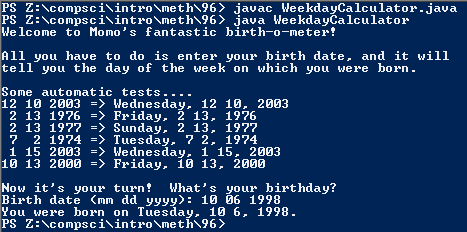# Assignemnt #104 Calling Methods From Other Files

## Code

```    ///Name: Morgan Kaplan
///Period: 5
///Project Name: CMFOF
///File Name: CMFOF.java

import java.util.Scanner;

public class CMFOF
{
public static void main( String[] args )
{
Scanner momo = new Scanner(System.in);

System.out.println("Welcome to Momo's fantastic birth-o-meter!");
System.out.println();
System.out.println("All you have to do is enter your birth date, and it will");
System.out.println("tell you the day of the week on which you were born.");
System.out.println();
System.out.println("Some automatic tests....");
System.out.println("12 10 2003 => " + WeekdayCalculator.weekday(12,10,2003));
System.out.println(" 2 13 1976 => " + WeekdayCalculator.weekday(2,13,1976));
System.out.println(" 2 13 1977 => " + WeekdayCalculator.weekday(2,13,1977));
System.out.println(" 7  2 1974 => " + WeekdayCalculator.weekday(7,2,1974));
System.out.println(" 1 15 2003 => " + WeekdayCalculator.weekday(1,15,2003));
System.out.println("10 13 2000 => " + WeekdayCalculator.weekday(10,13,2000));
System.out.println();

System.out.print("Birth date (mm dd yyyy): ");
int mm = momo.nextInt();
int dd = momo.nextInt();
int yyyy = momo.nextInt();

// put a function call for weekday() here

String birthday = WeekdayCalculator.weekday(mm,dd,yyyy) + ", " + MonthName.month_name(mm) + " " + dd + ", " + yyyy;

System.out.println("You were born on " + birthday + "!");
}

public static String weekday( int mm, int dd, int yyyy )
{
int yy, total;
String date = "";

// bunch of calculations go here

yy = yyyy - 1900;
total = yy / 4;
total = total + yy;
total = dd + total;
total = total + MonthOffset.monthOffset(mm);

if ( WeekdayCalculator.isLeap(yyyy) == true && MonthName.month_name(mm) == "January" || MonthName.month_name(mm) == "February" )
total = total - 1;

total = total % 7;

date = WeekdayName.weekday_name(total);

return date;
}

}
```

### Picture of Output